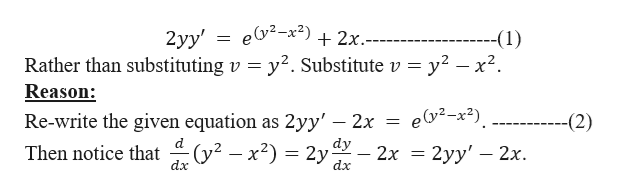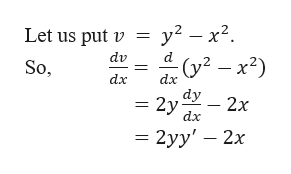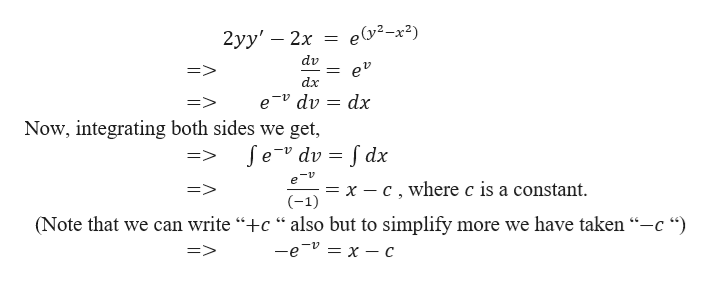# I am confused on this problem:2yy'=e^(y^2-x^2)+2xI tried to sub with v=y^2, but I don't understand how to separate the variable with e around.

Question
15 views

I am confused on this problem:

2yy'=e^(y^2-x^2)+2x

I tried to sub with v=y^2, but I don't understand how to separate the variable with e around.

check_circle

star
star
star
star
star
1 Rating
Step 1

We need to solve,help_outlineImage Transcriptionclose2yy' = ey2-x2)+2x.- Rather than substituting v y2. Substitute v --(1) y2 -x2 Reason: ey2-x2) Re-write the given equation 2yy' — 2х -(2) as dy Then notice that (y2 - x2) = 2y 2х 3D 2уу' — 2х. dx dx fullscreen
Step 2

So, to solve the given differential equation (2),help_outlineImage Transcriptionclosey2 -x2 (y2 -x2) Let us put v dv d So, dx dx dy 2y - 2x dx - 2уy' — 2х fullscreen
Step 3

Now, equation (2)...help_outlineImage Transcriptioncloseе(у?-х?) 2yy' — 2х dv => ev 11 dx e dv Now, integrating both sides we get, => dx fe dv dx ev = x - c,where c is a constant (-1) (Note that we can write "+c ** also but to simplify more we have taken "-c "") —е -v 3 х — с fullscreen

### Want to see the full answer?

See Solution

#### Want to see this answer and more?

Solutions are written by subject experts who are available 24/7. Questions are typically answered within 1 hour.*

See Solution
*Response times may vary by subject and question.
Tagged in

### Math